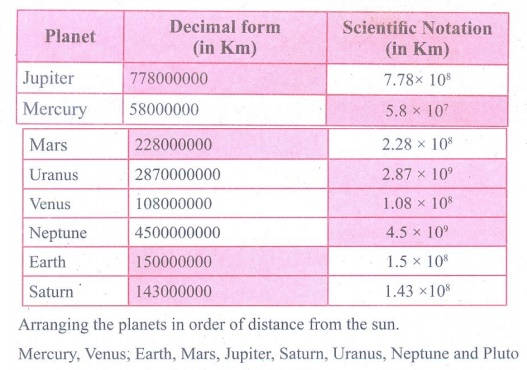Home | | Maths 9th std | Exercise 2.8: Scientific Notation

# Exercise 2.8: Scientific Notation

Maths : Real Numbers: Book Back, Exercise, Example Numerical Question with Answers, Solution : Exercise 2.8: Scientific Notation

Exercise 2.8

1. Represent the following numbers in the scientific notation:

(i) 569430000000 (ii) 2000.57 (iii) 0.0000006000 (iv) 0.00090000022. Write the following numbers in decimal form:

(i) 3. 459 ×106 (ii) 5. 678 ×104 (iii) 1. 00005 ×10−5 (iv) 2. 530009 ×10−73. Represent the following numbers in scientific notation:

(i) (300000)2 × (20000)4

(ii) (0. 000001)11 ÷ (0. 005)3

(iii) {( 0. 00003 )6 ×( 0 .00005)4 } ÷ {(0.009)3 ×(0.05)2 }4. Represent the following information in scientific notation:

(i) The world population is nearly 7000,000,000.

(ii) One light year means the distance 9460528400000000 km.

(iii) Mass of an electron is 0.000 000 000 000 000 000 000 000 000 00091093822 kg.5. Simplify:

(i) (2.75 × 107) + (1.23 × 108)

(ii) (1.598×1017) – (4.58 ×1015)

(iii) (1.02 × 1010) × (1.20 × 10–3)

(iv) (8.41 × 104) ÷ (4.3 × 105)Activity − 3

The following list shows the mean distance of the planets of the solar system from the Sun. Complete the following table. Then arrange in order of magnitude starting with the distance of the planet closest to the Sun.Solution:Tags : Numerical Problems with Answers, Solution | Real Numbers | Maths , 9th Maths : UNIT 2 : Real Numbers
Study Material, Lecturing Notes, Assignment, Reference, Wiki description explanation, brief detail
9th Maths : UNIT 2 : Real Numbers : Exercise 2.8: Scientific Notation | Numerical Problems with Answers, Solution | Real Numbers | Maths